﻿ Intersection of Sets | Calculators4U

# Intersection of Sets

In the set operations, let's calculate intersection of sets. The intersection of two sets A and B is the set that contains exactly all the elements that are in both A and B.

### Intersection of Sets

For two given sets A and B, A∩B (read as A intersection B) is the set of common elements that belong to set A and B. The number of elements in A∩B is given by n(A∩B) = n(A)+n(B)−n(A∪B), where n(X) is the number of elements in set X. To understand this set operation of the intersection of sets better, let us consider an example: If A = {1, 2, 3, 4} and B = {3, 4, 5, 6}, then the intersection of A and B is given by A ∩ B = {3, 4}.

### Set Operations Table

Set OperationVenn DiagramInterpretation
Union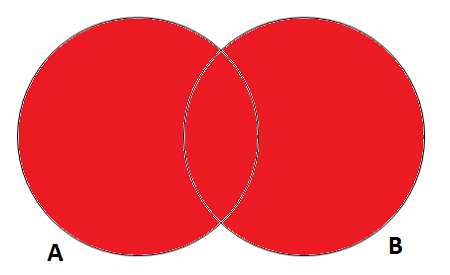A U B, is the set of all values that are a member of A, or B, or both.
Intersection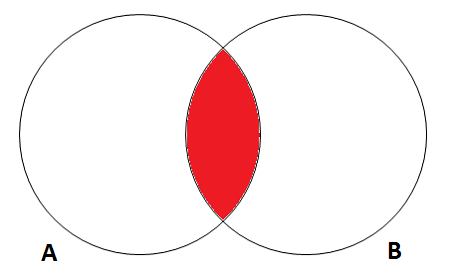A ∩ B, is the set of all values that are a member of both A and B.
Difference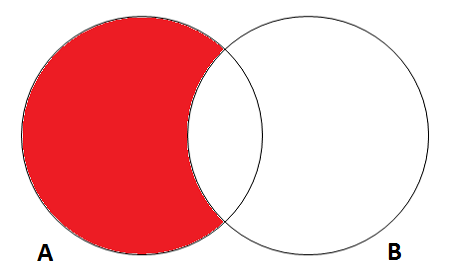A \ B, is the set of all values of A that are not members of B.
Symmetric Difference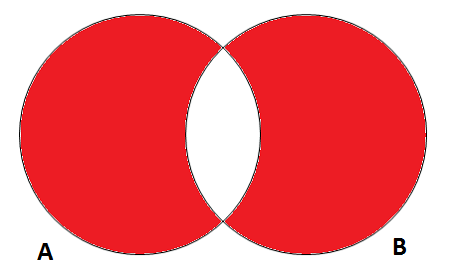A ∆ B, is the set of all values which are in one of the sets but not both.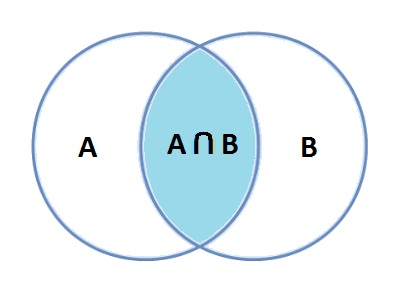### Spreading Knowledge Across the World

USA - United States of America  Canada  United Kingdom  Australia  New Zealand  South America  Brazil  Portugal  Netherland  South Africa  Ethiopia  Zambia  Singapore  Malaysia  India  China  UAE - Saudi Arabia  Qatar  Oman  Kuwait  Bahrain  Dubai  Israil  England  Scotland  Norway  Ireland  Denmark  France  Spain  Poland  and  many more....